The triangle

TYPE A TRIANGLE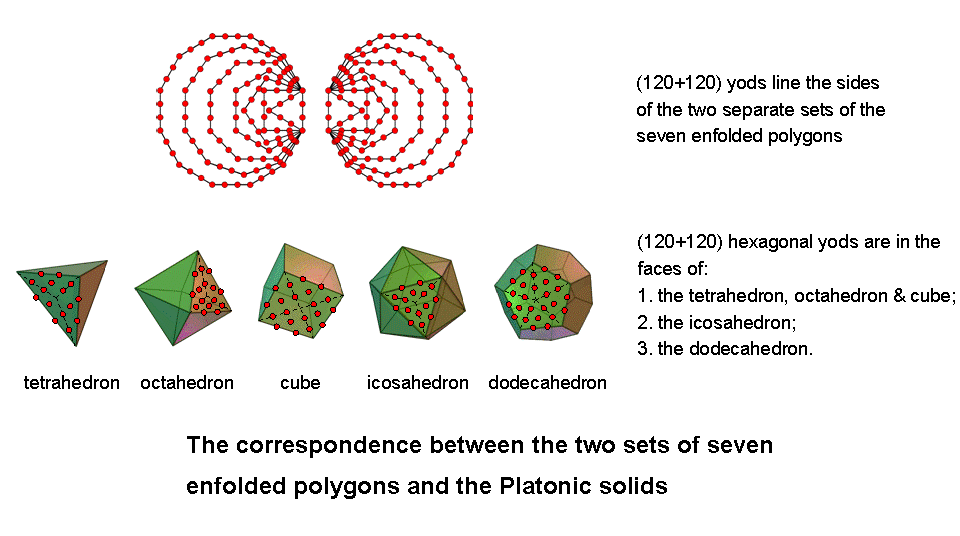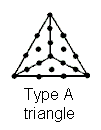(1) Number of yods = 19, which is the tenth odd integer (10 = fourth triangular number) and the eighth prime number (8 = fourth even integer). All integers are the sum of at most 19 fourth powers . This property would have delighted the early Pythagoreans in view of the paramount importance they attached to the number 4. The arithmetic mean of the first 19 triangular numbers is 70, which we saw in Sacred geometry is the number of corners of the (7+7) enfolded polygons of the inner Tree of Life (#13), the number of hexagonal yods in the 2nd-order tetractys (#35) and the number of yods in the outer Tree of Life when its 16 triangles are tetractyses (#36).

Two separate Type A triangles contain 38 yods. The arithmetic mean of the first 38 triangular numbers is 260, which we found in Sacred geometry to be the number of yods outside the root edge in seven enfolded Type A polygons (#26). Therefore, the triangle encodes arithmetically the number of corners of (7+7) enfolded polygons, whilst two triangles encode the population of yods in seven enfolded Type A polygons outside the root edge. This is a remarkable property of the tetractys-divided triangle.

(2) The number 19 is the ratio:where 28 is the second perfect number and the seventh triangular number: 28 = 1 + 2 + 3 + 4 + 5 + 6 + 7.
(3) Number of hexagonal yods = 15, which is the number of YAH, the Godname of Chokmah.
(4) 19th prime number = 67, the number of Binah. It is fitting that the simplest geometrical figure — the triangle — should embody the number of the Sephirah heading the Pillar of Judgement that embodies abstract archetypes of form.
(5) Two joined, Type A triangles contain 34 yods. As we saw in #9 of Human skeleton, this is the number of bones in the human axial skeleton that occur singly. It is also the number of corners of the sectors in the seven polygons above or below the centre of the disdyakis triacontahedron — the polyhedral version of the outer form of the Tree of Life (see here) — that are created by its vertices in planes perpendicular to an axis passing through two opposite C vertices.
(6) Sum of integers 1, 2, 3, ... 19 assigned to the 19 yods of the triangle = 190

 19 19 19 = 19 19 19 19 19 19 19 .

The 19th triangular number 190 is the only triangular number with the property of having such a tetractys representation, i.e., where 1 + 2 + ... + n = 10n = (1+2+3+4)n.

The Godname numbers of Kether and Chesed determine the number 190 embodied in a Type A triangle as follows: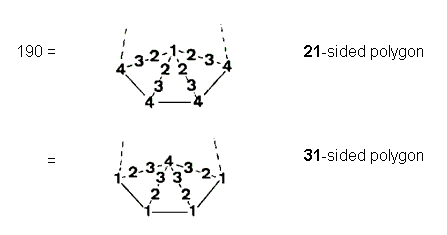whilst the sum of the first (19+19) integers assigned to the yods of two separate Type A triangles is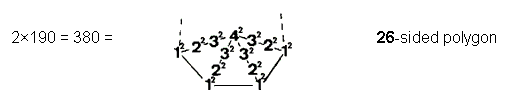The significance of the number 380 is that it is the number of yods contained in the 7-tree when its triangles are turned into tetractyses . In other words, the pair of triangles embody a number that is the yod population of the 7-tree mapping the physical plane, or space-time continuum. Also, 190 = 76 + 49 + 65, i.e., it is the sum of the Godname numbers of the three Sephiroth of Construction on the central Pillar of Equilibrium of the Tree of Life.

(7) The 10 odd integers and the 9 even integers up to 19 can be assigned to, respectively, the 10 yods inside the triangle and the 9 yods on its boundary with the properties: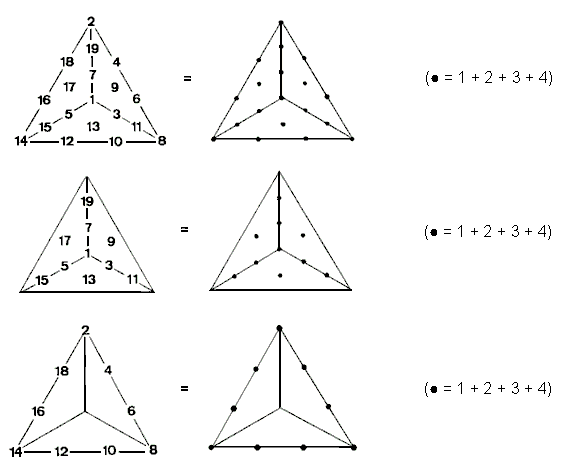The sum of the 9 odd integers surrounding the centre of the triangle is 99, which is the number of SLs on each side pillar of the 49-tree. This parameter of CTOL is thus encoded in the triangle. The sum of the 10 integers on the boundary and at the centre of the triangle is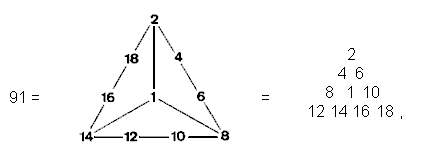which is the number of trees in CTOL. The central integer 1, which occupies the position of the yod corresponding to Malkuth, denotes the lowest tree (the most ‘Malkuth' level of CTOL), and the sum (90) of the surrounding nine even integers in either the triangle or the tetractys is the number of higher trees. This reminds us of Plato's Lambda tetractys array of 10 integers, whose ratios generate the tone ratios of the Pythagorean musical scale (see here). The significance of this is that the even integers on the boundary of the Type A triangle add up to 90, which is a parameter of all holistic systems. The sum of the three outer corner integers in the first representation of the number 91 is 24 (= 1×2×3×4), the sum of the remaining seven integers being 67, the number of Binah. The sum of the integers at all four corners is 25. The representation therefore marks out the 25-tree section of CTOL. This is prescribed by ADONAI MELEKH, the Godname of Malkuth with number value 155 because the 25-tree has 155 SLs. The 67th tree down in CTOL is the 25th from its base. It marks the last of the lowest 25 trees that bear a formal correspondence to the 25 spatial dimensions of the physical universe. The sum of the four integers at these corners defining the shapes of the tetractyses is the number of spatial dimensions in which matter extends.

The sum of the 15 integers at the centres & corners of the three hexagons of the Type A triangle is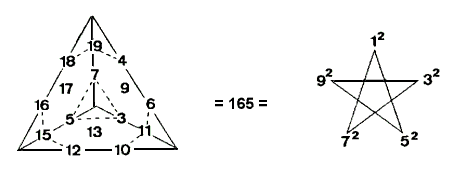The sum of the four integers at the corners of the sectors is 52. This demonstrates the beautiful arithmetic properties of the set of the first 19 integers when arranged according to the yods in a Type A triangle.
(8) Sum of the squares of the first 19 integers is

12 + 22 + 32 +… + 192 = 2470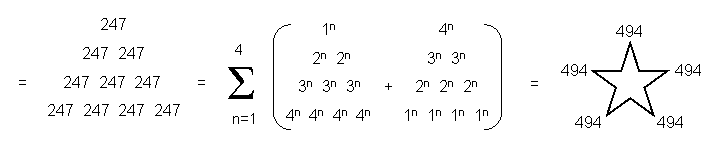where

247 = 26 + 31 + 76 + 49 + 65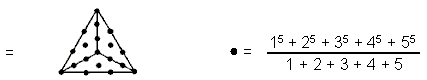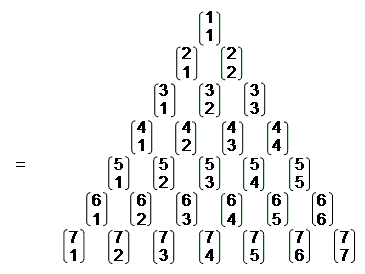(the first seven rows of the indefinitely-extending array of binary coefficients known as 'Pascal's Triangle') and

494 = 21 + 50 + 76 + 129 + 153 + 65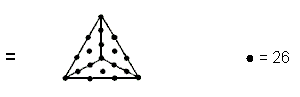11 21 31 41 12 22 32 42 = 13 23 33 43 14 24 34 44  .

(9) The sum of the squares of the 38 integers in a pair of triangles = 4940

 494 494 494 = 494 494 494 494 494 494 494 .

The number 247 generated by the squares of the first 19 integers is the number of yods other than corners in seven separate Type A polygons, 494 being the number of such yods in the (7+7) separate Type A polygons. Alternatively, 247 yods line their 48 tetractys sectors:

 247 =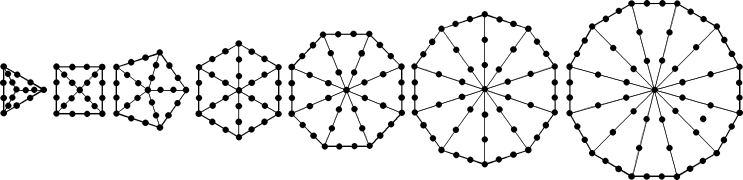We see that, in the sum of the squares that can be assigned to its 19 yods, the Type A triangle embodies another parameter of the seven polygons making up the inner Tree of Life. 2470 has the remarkable representation: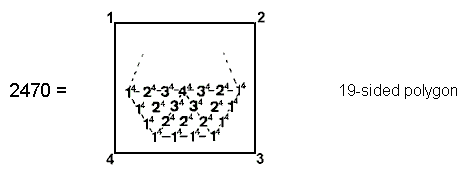It shows further how the number 19 generated by the tetractys-divided triangle determines the number 2470.

The significance of this number vis-à-vis CTOL is that it is the number of yods in 49 overlapping Trees of Life when their triangles are turned into tetractyses , these being the Tree of Life representation of the 49 subplanes of the seven planes making up the cosmic physical plane. The 1-tree contains 19 triangles . By embodying the number 19, the Type A triangle encodes the 1-tree, the 7-tree and the 49-tree. The 1-tree is the Malkuth level of the 7-tree, which is the analogous Malkuth level of the 49-tree. The Type A triangle therefore encodes the Malkuth levels of CTOL.

(10) The sum of the squares of the first 10 odd integers assigned to yods inside the Type A triangle is

 133 133 133 1330 = 133 133 133 133 133 133 133 .

1330 is the sum of the squares of the integers 2, 3 & 4 surrounding 12 at the centre of the 19-sided polygon: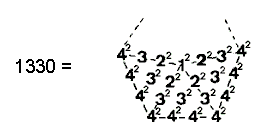This number is the number of yods in the 26-tree that is the higher counterpart of 26-dimensional space-time when its triangles are converted into tetractyses . In this way, the Type A triangle can be said to determine the 26 dimensions of the space-time of bosonic strings predicted by quantum mechanics! 1330 is also the sum of the first 19 triangular numbers. This is the mathematical connection between the Type A triangle and the dimensionality of space-time.

A single Tree of Life contains 70 yods when its triangles are converted into tetractyses. As 1330 = 19×70, we see that this number is the yod population of 19 separate trees. The number 133 is the dimension of the exceptional group E7, which is a subgroup of the superstring symmetry group E8.

(11) The four yods of the root edge, from which the triangle is the first polygon to be generated, can be represented by the four corners of a square. Assigning the integers 1, 2, 3 & 4 to these corners: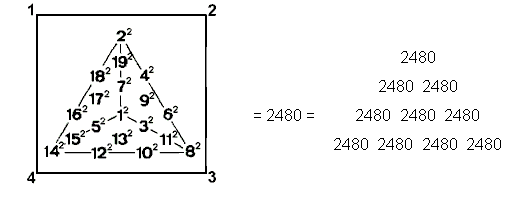The number 2480 is the number of yods in the 49-tree when its triangles are turned into tetractyses . It is the number of space-time components of the 248 gauge fields associated with E8. The Type A triangle encodes arithmetically the dimension 133 of E7, for this number is defined by the squares of the odd integers inside it. Together with the root edge, which symbolizes the Pythagorean integers 1, 2, 3 & 4, the triangle encodes the dimension of E8 as well. The 26-tree and the 49-tree are encoded in, respectively, the first five separate Type A polygons and all seven polygons and their root edge (see here). Their yod populations are the sums, respectively, of the squares of integers inside the Type A triangle and all its squares, including the integers 1, 2, 3 & 4 at the corners of the enclosing square. The difference in yod populations between the 49-tree and 49 overlapping Trees of Life = 2480 − 2470 = 10 yods. They correspond to the sum of the 1, 2, 3, 4 at the corners of the above square enclosing the  squares of the 19 integers in the Type A triangle.

(12) 19 is the number of combinations of three types of objects X, Y and Z taken one, two or three at a time: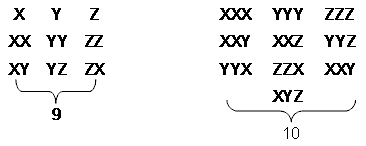Notice the one-to-one correspondence between these kinds of combinations and the types of yods in the tetractys-divided triangle: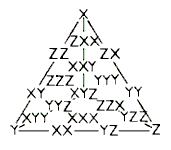The three single objects correspond to its corners, the nine combinations of one or two objects correspond to the nine yods on its boundary and the ten combinations of three objects correspond to the ten internal yods.

(13) The Type A triangle is a representation of the Godname number of Tiphareth when its 19 yods are either 4 or the squares of 1, 2, 3 & 4:Notice that the sum of the four corner integers in the first triangle is 19.

(14) A triangular array of integers 1, 2, 3 & 4 defines the number of Paths in the Tree of Life: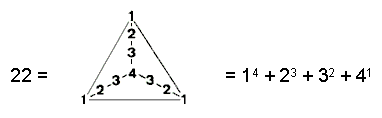A similar array of their fourth powers defines the number of SLs in CTOL:(15) ADONAI, the Godname of Malkuth, prescribes the 10-tree that is the counterpart of the 10-dimensional space-time of superstrings (see here). Malkuth of the 10th Tree is the 19th SL on the central pillar of CTOL. In addition, the 19th square number 192 = 361 is the number of vertices, lines & triangles (that is, geometrical elements) making up the 10-tree . The number 19 embodied in the Type A triangle determines in this way the dimensionality of superstring space-time.

(16) Kether of the 19th tree (Malkuth of the 21st tree) is the 41st SL on the central pillar of CTOL, where 41 is the 21st odd integer. 19 overlapping Trees have 40 SLs on this pillar. Assigning the 40 odd integers 3, 5, 7... 81 to these SLs, their sum:

1680 = 412 − 1 = 3 + 5 + 7... + 81,

is a number that quantifies the 3-dimensional structure of superstrings, being the number of circular turns of each helical whorl of the UPA described by C.W. Leadbeater (see here). As the simplest geometrical figure, the triangle embodies the number 19 that specifies a section of CTOL arithmetically encoding the form of the fundamental unit of matter — the subquark state of the E8×E8 heterotic superstring.

When the 232 triangles of 19 overlapping Trees are turned into tetractyses, they contain

 97 97 97 970 = 97 97 97 97 97 97 97 .

yods , where

97 = 21 + 26 + 50

is the sum of the Godname numbers of the Supernal Triad and the 49th odd integer, as well as the number value of Haniel, the Archangel of Netzach. It is remarkable that 970 is the sum of the number 474 of Daath “(Knowledge”) and the number 496 of Malkuth (“Kingdom”). We have just seen how the number 19 determines in an arithmetic way numbers characterizing fundamental aspects of superstring physics. We now find that the number value of “knowledge of Malkuth” defines the very set of Trees that encodes arithmetically the number 1680 characterizing the 3‑dimensional structure of superstrings — the Malkuth level of the Tree of Life in the subatomic world. Furthermore, 19 overlapping Trees have 620 yods other than SLs on their Paths when their triangles are converted into tetractyses, where 620 is the number of Kether .

(17) Since 192 − 1 = 360 = 3 + 5 + 7 +... + 37,

1 + 3(192 − 1) = 1 + 3(3 + 5 + 7 +... + 37) = 1 + 3×360 = 1081,

i.e., the number of Tiphareth is represented by a 3-fold array of 55 odd integers (55 is the tenth triangular number) comprising the first 19 odd integers.

(18) The equations: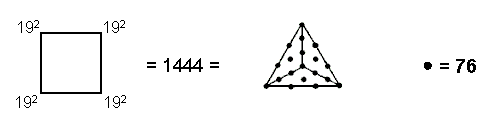where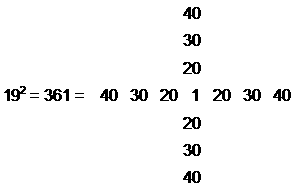express the fundamental connection between the number 19, the integers 1, 2, 3 & 4, the Tetrad (symbolized by the square) and the number 76 of YAHWEH ELOHIM, the Godname of Tiphareth. 1444 is the number of vertices & lines constituting the 65-tree . In other words, if each of the 65 SLs of the 10-tree that is the counterpart of 10-dimensional space-time and prescribed by the number 65 of the Godname ADONAI were regarded as a Tree of Life in itself, the minimum number of geometrical elements needed to construct the resulting 65-tree would be 1444. This number is also the number of yods other than centres in (7+7) separate, Type B polygons + root edge . Encoded in the 14 Type B polygons is the number of bits of information required to build the 65-tree, which is analogous to the 10‑tree. We see that the yod population of the two sets of seven Type B polygons separated by the root edge determines the 65-tree and therefore the 10-tree, which is a map of the 10‑dimensional space-time of superstrings. This is in turn encoded arithmetically in the triangle through the Tetrad because, when the number 4 is assigned to its 19 yods, their sum is 76 and, when the latter is assigned to the same yods, the resulting number is 1444.

TYPE B TRIANGLE(1) Number of yods = 46 = 15 + 31, i.e., the sum of the Godname numbers of Chokmah and Chesed on the Pillar of Mercy. Composite numbers are positive integers that have at least one positive divisor other than 1 and themselves, i.e., they are not prime numbers. Counting from the number 4 (the first composite number), 46 is the 31st composite number, where 31 is the number of EL, the Godname of Chesed. The 46th triangular number is 1081:

1081 = 1 + 2 + 3 +... + 46,

i.e., the Type B triangle defines the number of Tiphareth.

(2) The 46th odd integer is 91 — the number of Trees in CTOL. Just as Tiphareth is the centre of the Tree of Life, so Tiphareth of the 46th Tree (the 275th SL) is the halfway point in CTOL because 550 = 275 + 275. Hence, the Type B triangle arithmetically encodes both the number of Trees in CTOL and its centre.

(3) The 46th prime number is 199, which is a parameter of CTOL, being the number of stages of descent of the Lighting Flash from the top of the 49th Tree . Therefore, the Type B triangle encodes not only CTOL but also the section mapping the cosmic physical plane, namely, the 49-tree.

(4) Starting with 4 (the Pythagorean Tetrad), the sum of the 22 even integers up to 46:

4 + 6 + 8 +... + 46 = 550

is the number of SLs in CTOL (one can say that the Tree of Life with its 22 Paths encodes the number 550 if these numbers are assigned to them, starting with the Tetrad). Starting with 16 (= 42), the sum of the 16 even integers up to 46:

16 + 18 + 20 +... + 46 = 496

is the number of Malkuth and the dimension of the superstring symmetry groups SO(32) and E8×E8. These properties are represented by a Type B triangle with the number 16 at its centre, the next three numbers 18, 20 and 22 at its corners and the 12 even integers 24, 26... 46 along the sides of the three Type A triangles (shown below by thick lines):The number 16 is the number of simple roots of E8×E8, whilst the sum of the 15 other integers forming the boundaries of the Type A triangles is 480, which is the number of non-zero roots of E8×E8. The sum of the integers at their corners is

16 + 18 + 20 + 22 = 76,

which is the Godname number of Tiphareth. Hence, both the number of Tiphareth and its Godname number are represented in this Type B triangle, as well as the numbers 496 (sum of 16 integers on boundaries of Type A triangles) and 550 (sum of these 16 boundary integers and the central three-fold array of integers 4, 6, 8, 10, 12 & 14). The number 22 at the apex of the triangle is, of course, the number of Paths in the Tree of Life.

(5) The sum of the squares of the first 46 integers is

12 + 22 + 32 +... + 462 = 33511 = 31×1081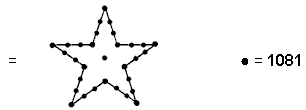The sum of the cubes of the first 46 integers is

13 + 23 + 33 +… + 463 = 10812.

(6) The sum of the first n triangular numbers is

T1 + T2 +… + Tn = n(n+1)(n+2)/6 = (n+2)Tn/3

(See note 13). For n = 46:

T1 + T2 +… + T46 = 16T46

 1081 1081 1081 = 1081 1081 1081 1081 1081 1081 1081

The number 46 is unique in that only the sum of the first 46 triangular numbers can be represented as a 4×4 square array of the 46th triangular number — the gematria number value of Tiphareth, meaning "beauty."
(7) Two separate Type B triangles have

 1 2 3 4 12 5 78 = 11 6 10 9 8 7

hexagonal yods, where 78 is the dimension of the exceptional Lie group E6. This is how the Tetrad defines through its symbol — the square — the symmetry group believed by many physicists to incorporate the physics of the Standard Model below the energy scale of grand unification described by E8×E8. Two enfolded, Type B triangles have 88 yods, 76 hexagonal yods and 84 yods outside the root edge, where 84 = 12 + 32 + 52 + 72. They embody a basic parameter of the inner Tree of Life because the seven enfolded polygons have 47 sectors with 88 sides. Separate Type A and Type B triangles contain 65 yods. Enfolded, they contain 61 yods, of which 50 are hexagonal yods outside the root edge. This compares with two enfolded Type A triangles, which contain 26 hexagonal yods outside the root edge. The Godname numbers of Chokmah and Binah are the numbers of yods corresponding to Sephiroth of Construction outside the root edge of, respectively, two enfolded Type A triangles and a Type A and a Type B triangle.

(8) The Type B triangle with 46 yods embodies the number of paired bones in the human axial skeleton and the number of chromosomes in the human cell, i.e., 46 is its diploid number.

TYPE C TRIANGLE
A Type C triangle has 127 yods:

 127 =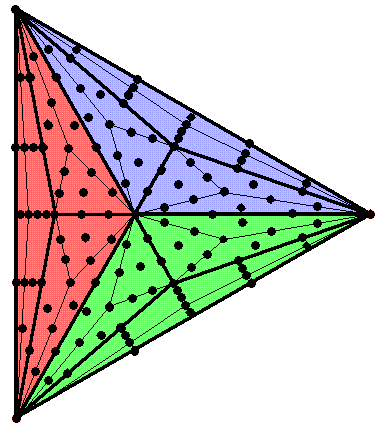127 is the 31st prime number, where 31 is the number value of EL ("God"), the Godname of Chesed. We saw in the discussion of the Type B triangle that it has 46 yods, where 46 is the 31st composite number. This shows how EL prescribes both Type B and Type C triangles. 123 yods are outside the left-hand side of a Type C triangle, where 123 is the tenth Lucas number L10 after the first one L0 = 2 and

L10 = Φ10 + Φ−10,

where Φ = (1+5)/2 = 1.618... is the Golden Ratio. This means that the pair of joined Type C triangles have 246 yods outside their shared side, where 246 is the number value of Gabriel, the Archangel of Yesod. Including the four yods in the shared side, the pair of separate, Type C triangles has 250 yods, that is, 248 yods surround the centres of the two Type C triangles. Alternatively, as this pair of triangles is part of the inner Tree of Life, they have 248 yods other than the left-hand and right-hand corners, which coincide with corners of triangles of the outer Tree of Life. In other words, the two Type C triangles contain 248 yods that are intrinsic to them:This is how the triangle — the most basic geometrical shape — embodies the dimension 248 of E8. The eight purple yods at the centre of each Type C triangle and its three sectors denote the eight simple roots of E8, the 72 black yods on the sides of the (9+9) Type A triangles and at their centres denote the 72 roots of E6, the rank-6, exceptional subgroup of E8 and the remaining 168 white yods (84 in each Type C triangle) denote the 168 other roots of E8.

TRIANGLE WITH 2ND-ORDER TETRACTYSES AS SECTORS
The 2nd-order tetractys contains 85 yods (15 corners & 70 hexagonal yods of 10 1st-order tetractyses). When the n sectors of an n-gon are 2nd-order tetractyses, the number of yods in the n-gon = 72n + 1, where "1" denotes the centre of the n-gon. The number of corners of the 10n 1st-order tetractyses = 10n + 1 and the number of hexagonal yods = 62n. The number 72 is the number of Chesed and 62 is the number of Tzadkiel, its Archangel. A triangle (3-gon) has 217 yods (216 yods surrounding its centre), of which 31 are corners of 30 1st-order tetractyses and 186 are hexagonal yods, where 31 is the number of EL, the Godname of Chesed.

Compare this with the seven enfolded, Type A polygons of the inner Tree of Life. They have 264 yods distributed in 47 tetractys sectors. Therefore, (264−47=217) yods line their sides. Of these, one yod is the topmost corner of the hexagon, which coincides with the lowest corner of the hexagon enfolded in the next higher, overlapping Tree of Life, leaving 216 boundary yods that are intrinsic to the set of seven polygons enfolded in each Tree. The triangle with 2nd-order tetractyses as its sectors embodies the number of yods that shape the 47 tetractyses forming the seven enfolded polygons:The triangle with 2nd-order tetractyses as its sectors embodies the number of yods lining the 47 1st-order tetractyses in the 7 enfolded, Type A polygons of the inner Tree of Life.

A more detailed analysis of the correspondences between the inner Tree of Life and the triangle with 2nd-order tetractys sectors is discussed here.

Notes
1. The Penguin Dictionary of Curious and Interesting Numbers, David Wells (Penguin Books, 1988), p. 97.

2. Proof: the number of yods in the n-tree with its (12n+7) triangles turned into tetractyses = 50n + 30. The 7-tree has (7×50 + 30 = 380) yods in its 91 tetractyses.

3. Proof: the number of yods in n overlapping Trees of Life with their (12n+4) triangles turned into tetractyses = 50n + 20. 49 overlapping Trees have (49×50 + 20 = 2470) yods.

4. Proof: the number of triangles in the n-tree = 12n + 7. The 1-tree has (12+7=19) triangles.

5. Proof: using the formula given in (2), the number of yods in the 26-tree = 50×26 + 30 = 1330.

6. Proof: using the formula given in (2), the number of yods in the 49-tree = 50×49 + 30 = 2480.

7. Proof: the n-tree has (12n+7) triangles with (6n+5) corners and (16n+9) sides. Number of corners, sides & triangles in the n-tree = 34n + 21. The 10-tree has (10×34 + 21 = 361) corners, sides & triangles.

8. Proof: using the formula given in (3), the number of yods in 19 overlapping Trees = 50×19 + 20 = 970.

9. The number of Paths in n overlapping Trees of Life = 16n + 6. Two hexagonal yods lie on each Path when their triangles become tetractyses. 19 overlapping Trees have (16×19 + 6= 310) Paths with (2×310 = 620) hexagonal yods on them.

10. Using the formulae given in (7), the 65-tree has (6×65 + 5= 395) corners and (16×65 + 9 = 1049) sides, i.e., 1444 corners & sides.

11. The number of yods surrounding the centre of a Type B n-gon = 15n. Surrounding the centres of the seven separate Type B polygons are (15n = 15×48 = 720) yods. The number of yods in the two sets of polygons and the separate root edge = 2×720 + 4 = 1444.

12. The number of stages of descent of the Lightning Flash from the top of the n-tree = 4n + 3. The Lightning Flash descends from the top of the 49-tree in (4×49 + 3 = 199) stages.

13. The rth triangular number Tr = 1 + 2 + 3 + ... + r = ½r(r+1). Therefore, T1 + T2 + ... + Tn = ½ r2 + ½ r = n(n+1)(2n+1)/12 + n(n+1)/4 = n(n+1)(2n+1)/6.

Home